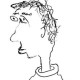# Two questions and a story$\bullet$ Let$f$ be some cuspidal Hecke eigenform, with associated Galois representation$\rho_{f}:G_{\mathbf{Q}}\to \mathrm{GL}_2(\overline{\mathbf{Q}_p})$. A notorious conjecture of Greenberg asserts that if$\rho_{f}|G_{\mathbf{Q}_p}$ is abelian (i.e. is a direct sum of characters), then$f$ is a CM form, or equivalently$\rho_f$ is induced from a character. At some point I was talking about this with Barry Mazur, and he suggested a possible generalization:

Let$\rho:G_{\mathbf{Q}} \to \mathrm{GL}_n(\overline{\mathbf{Q}_p})$ be an irreducible geometric Galois representation. Suppose that$\rho|G_{\mathbf{Q}_p}$ is a direct sum of characters. Is$\rho$ induced from a character?

Emerton found a nice argument which proves Greenberg’s conjecture conditionally on some kind of$p$-adic variational Hodge conjecture. Is there any similar evidence for this question in some higher-dimensional cases?$\bullet$ Do separated etale maps of schemes satisfy effective descent with respect to fpqc covers? This is known if one restricts to quasi-compact separated etale maps. An analogous result is true for perfectoid spaces.$\bullet$ Here’s a funny story I heard from Glenn Stevens a while back:

At some point in the early ’90s, before he announced his proof of Fermat, Wiles came to Boston and gave a seminar talk at BU. He spoke about what is now known as the Greenberg-Wiles duality formula. However, he didn’t mention his main motivations for this formula. The upshot is that Stevens came away from the talk with the sad feeling that Wiles had lost his touch.

## 3 thoughts on “Two questions and a story”

1.galoisrepresentations says:

You might want to impose a regularity condition. Any representation with finite image (non-reduced or not) will admit lots of primes for which the local representation is a direct sum of characters (or even trivial).

Like

1.arithmetica says:

Good point!

Like